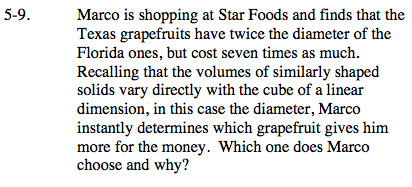### Home > PC > Chapter 5 > Lesson 5.1.1 > Problem5-9

5-9.What is the volume of the Florida grapefruit?

What is the radius of the Texas grapefruit in terms of the Florida radius?

Find T(x).

So which is the better deal?

$F(x)=\frac{4}{3}\pi r^3$

2r

$T(x)=\frac{4}{3}\pi(2r)^3$

$T(x)=(8)\frac{4}{3}\pi r^3$